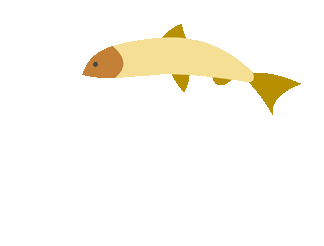top of page## Graph Games

##### Creating Graphs (Resources)
1. Understanding Graphs

This is a very good interactive way to see if you understand what information is in a graph.

##### Bar Graph (Resources)
###### Bar Graphs
1. Interactive Bar Graph

###### Double Bar Graphs
1. Explore Double Bar Graphs

Compare the difference in population of two different places.

2. Double Bar Graph Maker

##### Bar Graph (Games)
1. Bar Graph Quiz

See if you can answer the questions correctly.

##### Line Graph (Resources)
1. Math is Fun

Simple notes about what line graphs are and how they can be used.

##### Line Graph (Games)
1. Line Graph Interpretation

Test your knowledge with this online quiz.

##### Making Predictions (Resources)
1. Hamblen County School Video

Guided sample questions about making conjectures and predictions based on data.

1. BBC Examples

##### Biased Samples Graphs (Resources)
1. IXL Math

See how well you do with determining if the samples are biased or not.bottom of page